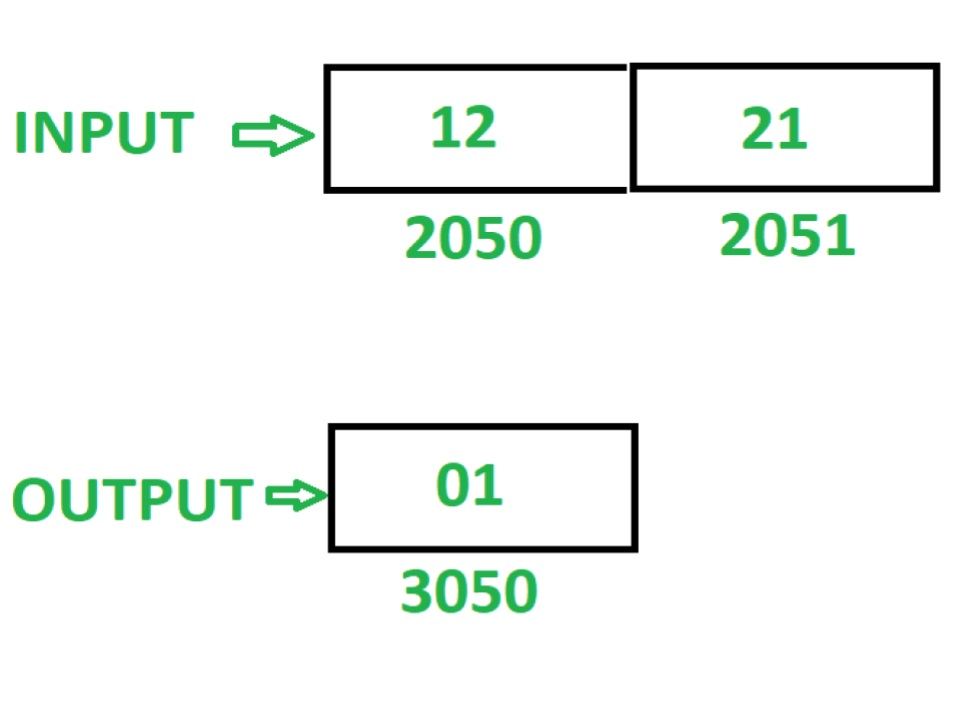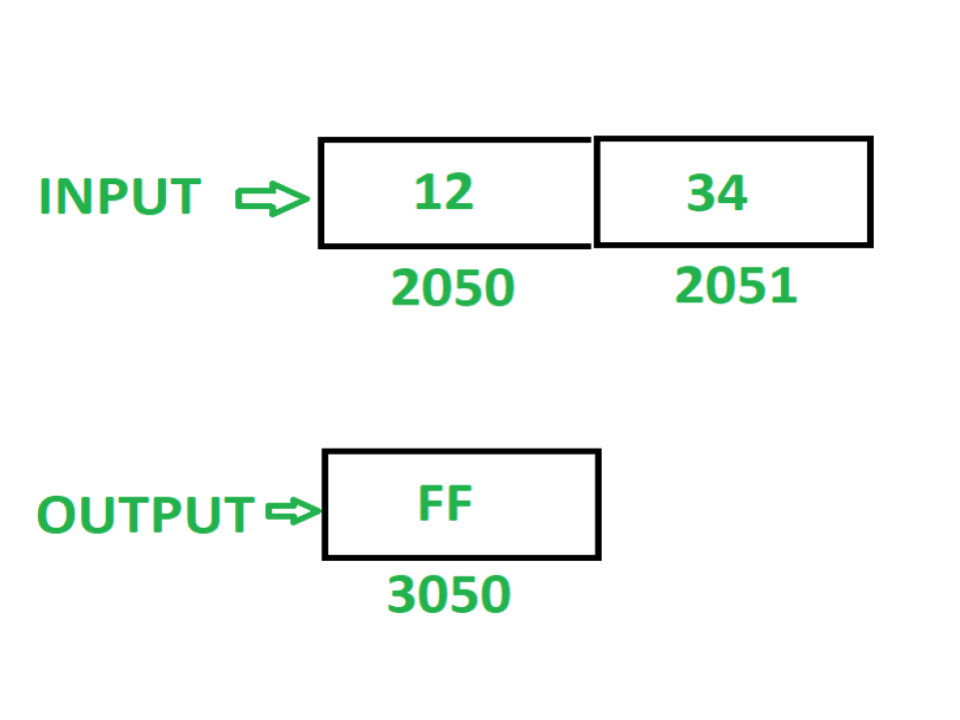GeeksforGeeks App
Open AppBrowser
Continue

# 8085 program to check whether the given 16 bit number is palindrome or not

Problem – Write an assembly language program to check whether the given 16 bit number is palindrome or not. If number is palindrome then store 01 at memory location 3050 otherwise store FF at memory location 3050.

Note – A palindrome number is a number that remains the same when its digits are reversed.

Assume that 16 bit number, to check for palindrome is stored at memory location 2050.

Examples –Algorithm –

1. Load contents of memory location 2050 in register L and contents of memory location 2051 in register H
2. Move contents of L in accumulator A
3. Reverse the contents of A by executing RLC instruction 4 times
4. Move the contents of A in L
5. Move the contents of H in A
6. Reverse the contents of A by executing RLC instruction 4 times
7. Move the contents of L in H
8. Move the contents of A in L
9. Store the content of L in memory location 2070 and contents of H in memory location 2071
10. Load the content of memory location 2050 in A
11. Move the content of A in register B
12. Load the content of memory location 2070 in A
13. Compare content of A and B. If the content is not same then store FF in A and store it in memory location 3050
14. If contents of A and B are same, then Load the content of memory location 2051 in A
15. Move the content of A in B
16. Load the content of memory location 2071 in A
17. Compare content of A and B. If the content is not same then store FF in A and store it in memory location 3050
18. If contents of A and B are same, then store 01 in A and store it in memory location 3050

Program –

2000LHLD 2050L <- M, H <- M
2003MOV A, LA <- L
2004RLCRotate accumulator content left by 1 bit without carry
2005RLCRotate accumulator content left by 1 bit without carry
2006RLCRotate accumulator content left by 1 bit without carry
2007RLCRotate accumulator content left by 1 bit without carry
2008MOV L, AL <- A
2009MOV A, HA <- H
200ARLCRotate accumulator content left by 1 bit without carry
200BRLCRotate accumulator content left by 1 bit without carry
200CRLCRotate accumulator content left by 1 bit without carry
200DRLCRotate accumulator content left by 1 bit without carry
200EMOV H, LH <- L
200FMOV L, AL <- A
2010SHLD 2070M <- L, M <- H
2013LDA 2050A <- M
2016MOV B, AB <- A
2017LDA 2070A <- M
201ACMP BA – B
201BJZ 2024Jump if ZF = 0
201EMVI A, FFA <- 01
2020STA 3050M <- A
2023HLTEND
2024LDA 2051A <- M
2027MOV B, AB <- A
2028LDA 2071A <- M
202BCMP BA – B
202CJZ 2035Jump if ZF = 0
202FMVI A, FFA <- FF
2031STA 3050M <- A
2034HLTEND
2035MVI A, 01A <- 01
2037STA 3050M <- A
203AHLTEND

Explanation – Registers A, H, L, B are used for general purpose.

1. LHLD 2050: loads contents of memory location 2050 in L and 2051 in H.
2. MOV A, L: moves content of L in A.
3. RLC: shift the content of A left by one bit without carry. Repeat the current instruction 4 times so that contents of A get reversed.
4. MOV L, A: moves the content of A in L.
5. MOV A, H: moves the content of H in A.
6. RLC: shift the content of A left by one bit without carry. Repeat the current instruction 4 times so that contents of A get reversed.
7. MOV H, L: moves the content of L in H.
8. MOV L, A: moves the content of A in L.
9. SHLD 2070: stores the content of L in 2070 and H in 2071.
10. LDA 2050: load the content of memory location 2050 in A.
11. MOV B, A: moves the content of A in B.
12. CMP B: compares the content of A and B. It set the zero flag if content is same otherwise reset.
13. JZ 2024: jump to memory location 2024 if ZF = 1.
14. MVI A, FF: store FF in A.
15. STA 3050: store content of A in 3050.
16. HLT: stops executing the program and halts any further execution.
17. LDA 2051: load the content of memory location 2050 in A.
18. MOV B, A: moves the content of A in B.
19. LDA 2071: load the content of memory location 2071 in A.
20. CMP B: compares the content of A and B. It set the zero flag if content is same otherwise reset.
21. JZ 2035: jump to memory location 2035 if ZF = 1.
22. MVI A, FF: store FF in A.
23. STA 3050: store content of A in 3050.
24. HLT: stops executing the program and halts any further execution.
25. MVI A, 01: store 01 in A.
26. STA 3050: store content of A in 3050.
27. HLT: stops executing the program and halts any further execution.

My Personal Notes arrow_drop_up